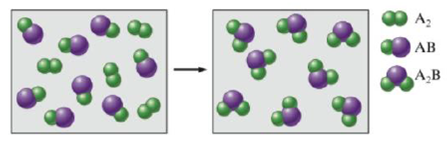Chapter 3, Problem 7RQ

Chapter
Section
Textbook Problem

Consider the hypothetical reaction between A2 and AB pictured below.What is the balanced equation? lf 2.50 moles of A2 are reacted with excess AB, what amount (moles) of product will form? If the mass of AB is 30.0 u and the mass of A2 are 40.0 u, what is the mass of the product? If 15.0 g of AB is reacted, what mass of A2 is required to react with all of the AB, and what mass of product is formed?

Interpretation Introduction

Interpretation: The balanced equation for the stated hypothetical reaction is to be determined. The mass of the various products formed in the given reactions is to be calculated.

Concept introduction: A chemical equation that satisfies the law of conservation of mass is known as a balanced chemical equation. That is the mass of the reactants is equal to the mass of the reactants formed.

To determine: A balance chemical equation for the given hypothetical reaction and the mass of the products formed in various other stated reactions.

Explanation

The green spheres are represented by A and the violet spheres by B .

The reaction obtained will be,

A2+ABA2B

But according to the law of conservation of mass, the mass always remains conserved.

Hence, the amount of an element present in the reactant side will be equal to the amount in the product side. Hence, for the given reaction, the coefficient 2 is added to AB on the reactant side and A2B on the product side for it to follow the law of conservation of mass. Therefore, a balance chemical equation for the given hypothetical reaction is,

A2+2AB2A2B .

To determine: The mass of the products formed when 2.5moles of A2 react with excess of AB .

The reaction that takes place is,

A2+2AB2A2B

One mole of A2 reacts with two moles of AB to give two moles of A2B .

Hence 2.5moles of A2 will react with 5moles of AB to give 5moles_ of A2B .

To determine: The mass of the products formed when 40.0u of A2 react with 30.0u of AB .

The reaction that takes place is,

A2+2AB2A2B

Mass of AB=30.0u

Mass of A2=40.0u

Hence, mass of A=20.0u

Since, the mass of AB is 30.0u and the mass of A is 20u , the mass of B is (3020)=10u .

The mass of the product, A2B formed is =2(A2+B)=2((40.0)+10.0)u=100u_

To determine: The mass of A2 required to react with all of AB and the mass of the products formed when 15g of AB is reacted

Still sussing out bartleby?

Check out a sample textbook solution.

See a sample solution

The Solution to Your Study Problems

Bartleby provides explanations to thousands of textbook problems written by our experts, many with advanced degrees!

Get Started

Find more solutions based on key concepts